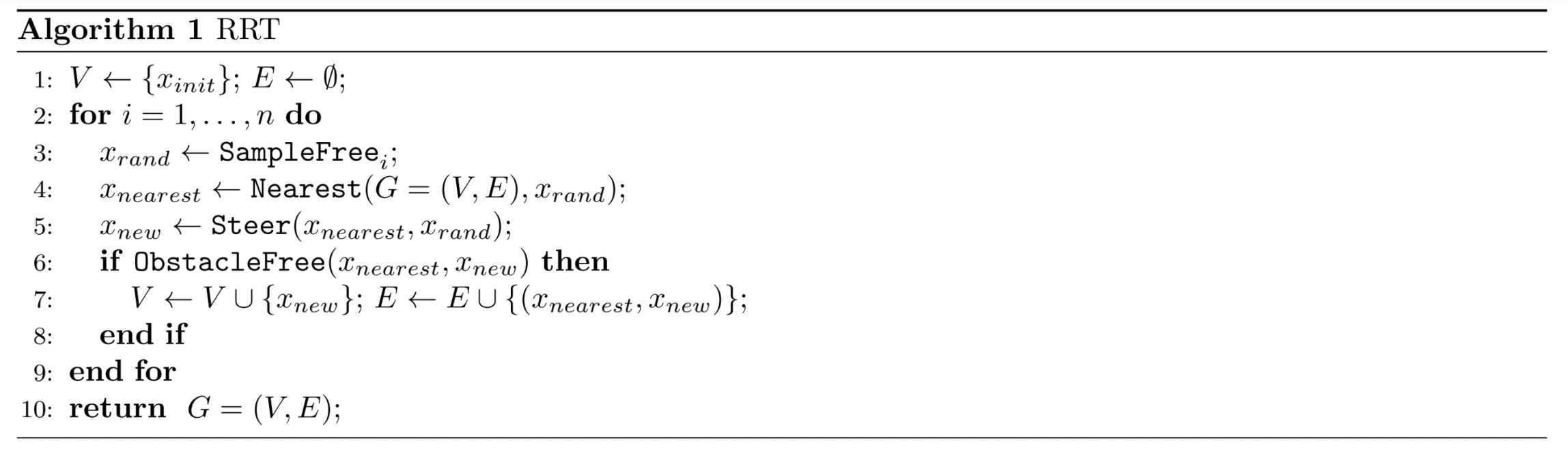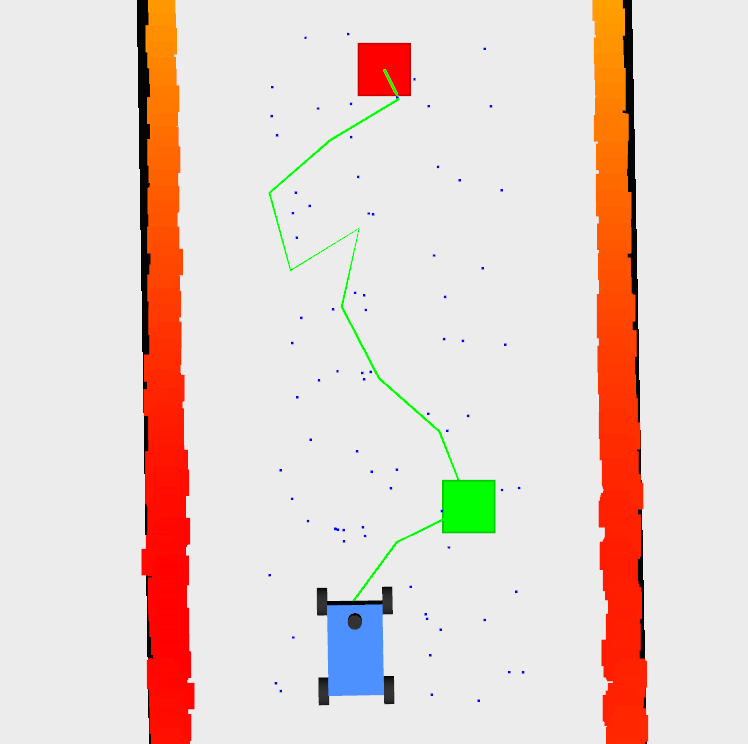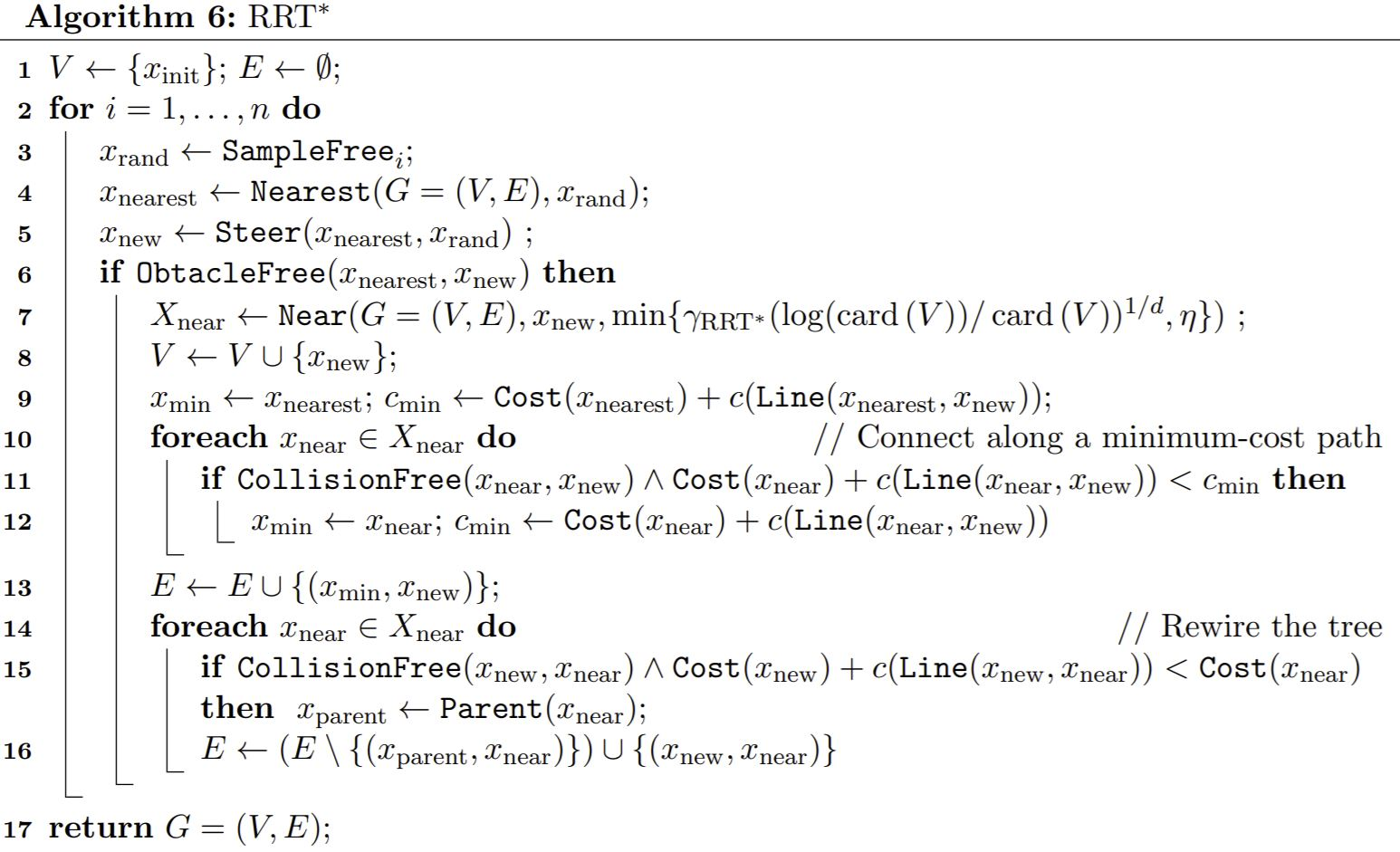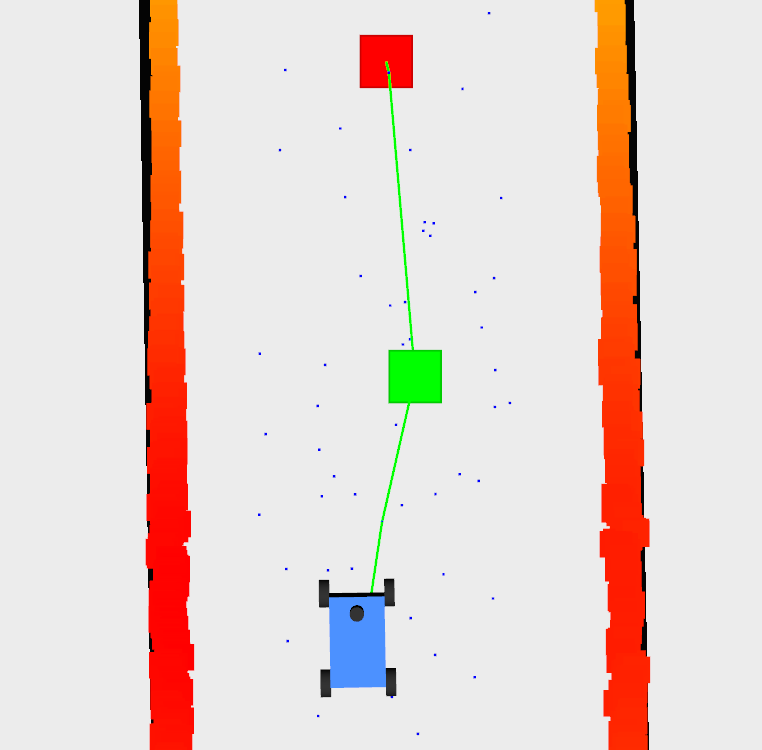# Lejun's Blog

'22 MSE in Robotics @ Penn
'20 BSE in MechE, Minor in EE @ UMich
'20 BSE in ECE @ SJTU

0%

During the Spring 2021 semester, I joined the F1Tenth team at Penn to conduct an interest project on multi-vehicle coordination. Lab 7 of the “F1Tenth” course is focused on RRT and RRT* that could be fundamental to my project, so I have completed it and will demonstrate below.

# Overview

This post contains my results for Lab 7, where the RRT and RRT* algorithm for path planning is implemented for the race car so that it can generate a set of desired waypoints to the goal. The Pure Pursuit algorithm in lab 6 is used for tracking the corresponding generated waypoints. They together allows the car to complete a loop of the Levine Hall smoothly. See the video below for a demonstration of the RRT algorithm:

# Demonstration of RRT

In the video, the red point is the goal point manually set according to the location of the car in the map; the blue points are the sampled points from the RRT algorithm; the green trajectory is the path found by the RRT algorithm; and the green point is the waypoint the car currently is following (so that the car can follow the green trajectory). Improvements were made that resulted in an implementation of the RRT* algorithm, which will be demonstrated later in this post.

# Procedure

The first step of the RRT algorithm is to define the goal point (the red point in the above figure). I defined them according to the current location of the car in the map. The algorithm then iteratively samples random point in a designated region (also defined according to the current location of the car in the map). A tree is maintained through this process and each iteration steers a node of the tree (the closest node) to the direction of the sampled point. The process is terminated when either the goal point is reached by the tree or the desired maximum number of iterations is reached. If the goal point is reached, we can then trace back the tree to find the desired trajectory. A pseudo code of the algorithm is presented below:RRT pseudo code.

A clear disadvantage of this algorithm is its suboptimality. The generated paths are non-smooth and hard to follow by the race cars. See the figure below,Non-smooth path generated by RRT.

A solution to this is to use the RRT* algorithm, which is an algorithm built on RRT, but rewiring the tree while adding new nodes to it, a pseudo code of the algorithm is presented below:[RRT* pseudo code.](https://arxiv.org/pdf/1105.1186.pdf)

The result of the RRT* algorithm is shown below for comparison to the result of RRT, a video demonstration is also shown in the next section:Smoooth path generated by RRT*.

More technical details can be found in the below prompt document and this paper:

My source codes for this lab can be found on github through this link.

# Demonstration of RRT*

The generated path is obviously smoother with the RRT*.

# Reference

The lecture video can be found below: